﻿

## Function score queryedit

The `function_score` allows you to modify the score of documents that are retrieved by a query. This can be useful if, for example, a score function is computationally expensive and it is sufficient to compute the score on a filtered set of documents.

To use `function_score`, the user has to define a query and one or more functions, that compute a new score for each document returned by the query.

`function_score` can be used with only one function like this:

```response = client.search(
body: {
query: {
function_score: {
query: {
match_all: {
}
},
boost: '5',
random_score: {
},
boost_mode: 'multiply'
}
}
}
)
puts response```
```res, err := es.Search(
"query": {
"function_score": {
"query": {
"match_all": {}
},
"boost": "5",
"random_score": {},
"boost_mode": "multiply"
}
}
}`)),
es.Search.WithPretty(),
)
fmt.Println(res, err)```
```GET /_search
{
"query": {
"function_score": {
"query": { "match_all": {} },
"boost": "5",
"random_score": {},
"boost_mode": "multiply"
}
}
}```
 See Function score for a list of supported functions.

Furthermore, several functions can be combined. In this case one can optionally choose to apply the function only if a document matches a given filtering query

```response = client.search(
body: {
query: {
function_score: {
query: {
match_all: {
}
},
boost: '5',
functions: [
{
filter: {
match: {
test: 'bar'
}
},
random_score: {
},
weight: 23
},
{
filter: {
match: {
test: 'cat'
}
},
weight: 42
}
],
max_boost: 42,
score_mode: 'max',
boost_mode: 'multiply',
min_score: 42
}
}
}
)
puts response```
```res, err := es.Search(
"query": {
"function_score": {
"query": {
"match_all": {}
},
"boost": "5",
"functions": [
{
"filter": {
"match": {
"test": "bar"
}
},
"random_score": {},
"weight": 23
},
{
"filter": {
"match": {
"test": "cat"
}
},
"weight": 42
}
],
"max_boost": 42,
"score_mode": "max",
"boost_mode": "multiply",
"min_score": 42
}
}
}`)),
es.Search.WithPretty(),
)
fmt.Println(res, err)```
```GET /_search
{
"query": {
"function_score": {
"query": { "match_all": {} },
"boost": "5",
"functions": [
{
"filter": { "match": { "test": "bar" } },
"random_score": {},
"weight": 23
},
{
"filter": { "match": { "test": "cat" } },
"weight": 42
}
],
"max_boost": 42,
"score_mode": "max",
"boost_mode": "multiply",
"min_score": 42
}
}
}```
 Boost for the whole query. See Function score for a list of supported functions.

The scores produced by the filtering query of each function do not matter.

If no filter is given with a function this is equivalent to specifying `"match_all": {}`

First, each document is scored by the defined functions. The parameter `score_mode` specifies how the computed scores are combined:

 `multiply` scores are multiplied (default) `sum` scores are summed `avg` scores are averaged `first` the first function that has a matching filter is applied `max` maximum score is used `min` minimum score is used

Because scores can be on different scales (for example, between 0 and 1 for decay functions but arbitrary for `field_value_factor`) and also because sometimes a different impact of functions on the score is desirable, the score of each function can be adjusted with a user defined `weight`. The `weight` can be defined per function in the `functions` array (example above) and is multiplied with the score computed by the respective function. If weight is given without any other function declaration, `weight` acts as a function that simply returns the `weight`.

In case `score_mode` is set to `avg` the individual scores will be combined by a weighted average. For example, if two functions return score 1 and 2 and their respective weights are 3 and 4, then their scores will be combined as `(1*3+2*4)/(3+4)` and not `(1*3+2*4)/2`.

The new score can be restricted to not exceed a certain limit by setting the `max_boost` parameter. The default for `max_boost` is FLT_MAX.

The newly computed score is combined with the score of the query. The parameter `boost_mode` defines how:

 `multiply` query score and function score is multiplied (default) `replace` only function score is used, the query score is ignored `sum` query score and function score are added `avg` average `max` max of query score and function score `min` min of query score and function score

By default, modifying the score does not change which documents match. To exclude documents that do not meet a certain score threshold the `min_score` parameter can be set to the desired score threshold.

For `min_score` to work, all documents returned by the query need to be scored and then filtered out one by one.

The `function_score` query provides several types of score functions.

### Script scoreedit

The `script_score` function allows you to wrap another query and customize the scoring of it optionally with a computation derived from other numeric field values in the doc using a script expression. Here is a simple sample:

```GET /_search
{
"query": {
"function_score": {
"query": {
"match": { "message": "elasticsearch" }
},
"script_score": {
"script": {
"source": "Math.log(2 + doc['my-int'].value)"
}
}
}
}
}```

In Elasticsearch, all document scores are positive 32-bit floating point numbers.

If the `script_score` function produces a score with greater precision, it is converted to the nearest 32-bit float.

Similarly, scores must be non-negative. Otherwise, Elasticsearch returns an error.

On top of the different scripting field values and expression, the `_score` script parameter can be used to retrieve the score based on the wrapped query.

Scripts compilation is cached for faster execution. If the script has parameters that it needs to take into account, it is preferable to reuse the same script, and provide parameters to it:

```GET /_search
{
"query": {
"function_score": {
"query": {
"match": { "message": "elasticsearch" }
},
"script_score": {
"script": {
"params": {
"a": 5,
"b": 1.2
},
"source": "params.a / Math.pow(params.b, doc['my-int'].value)"
}
}
}
}
}```

Note that unlike the `custom_score` query, the score of the query is multiplied with the result of the script scoring. If you wish to inhibit this, set `"boost_mode": "replace"`

### Weightedit

The `weight` score allows you to multiply the score by the provided `weight`. This can sometimes be desired since boost value set on specific queries gets normalized, while for this score function it does not. The number value is of type float.

`"weight" : number`

### Randomedit

The `random_score` generates scores that are uniformly distributed from 0 up to but not including 1. By default, it uses the internal Lucene doc ids as a source of randomness, which is very efficient but unfortunately not reproducible since documents might be renumbered by merges.

In case you want scores to be reproducible, it is possible to provide a `seed` and `field`. The final score will then be computed based on this seed, the minimum value of `field` for the considered document and a salt that is computed based on the index name and shard id so that documents that have the same value but are stored in different indexes get different scores. Note that documents that are within the same shard and have the same value for `field` will however get the same score, so it is usually desirable to use a field that has unique values for all documents. A good default choice might be to use the `_seq_no` field, whose only drawback is that scores will change if the document is updated since update operations also update the value of the `_seq_no` field.

It was possible to set a seed without setting a field, but this has been deprecated as this requires loading fielddata on the `_id` field which consumes a lot of memory.

```response = client.search(
body: {
query: {
function_score: {
random_score: {
seed: 10,
field: '_seq_no'
}
}
}
}
)
puts response```
```res, err := es.Search(
"query": {
"function_score": {
"random_score": {
"seed": 10,
"field": "_seq_no"
}
}
}
}`)),
es.Search.WithPretty(),
)
fmt.Println(res, err)```
```GET /_search
{
"query": {
"function_score": {
"random_score": {
"seed": 10,
"field": "_seq_no"
}
}
}
}```

### Field Value factoredit

The `field_value_factor` function allows you to use a field from a document to influence the score. It’s similar to using the `script_score` function, however, it avoids the overhead of scripting. If used on a multi-valued field, only the first value of the field is used in calculations.

As an example, imagine you have a document indexed with a numeric `my-int` field and wish to influence the score of a document with this field, an example doing so would look like:

```GET /_search
{
"query": {
"function_score": {
"field_value_factor": {
"field": "my-int",
"factor": 1.2,
"modifier": "sqrt",
"missing": 1
}
}
}
}```

Which will translate into the following formula for scoring:

`sqrt(1.2 * doc['my-int'].value)`

There are a number of options for the `field_value_factor` function:

 `field` Field to be extracted from the document. `factor` Optional factor to multiply the field value with, defaults to `1`. `modifier` Modifier to apply to the field value, can be one of: `none`, `log`, `log1p`, `log2p`, `ln`, `ln1p`, `ln2p`, `square`, `sqrt`, or `reciprocal`. Defaults to `none`.
Modifier Meaning

`none`

Do not apply any multiplier to the field value

`log`

Take the common logarithm of the field value. Because this function will return a negative value and cause an error if used on values between 0 and 1, it is recommended to use `log1p` instead.

`log1p`

Add 1 to the field value and take the common logarithm

`log2p`

Add 2 to the field value and take the common logarithm

`ln`

Take the natural logarithm of the field value. Because this function will return a negative value and cause an error if used on values between 0 and 1, it is recommended to use `ln1p` instead.

`ln1p`

Add 1 to the field value and take the natural logarithm

`ln2p`

Add 2 to the field value and take the natural logarithm

`square`

Square the field value (multiply it by itself)

`sqrt`

Take the square root of the field value

`reciprocal`

Reciprocate the field value, same as `1/x` where `x` is the field’s value

`missing`
Value used if the document doesn’t have that field. The modifier and factor are still applied to it as though it were read from the document.

Scores produced by the `field_value_score` function must be non-negative, otherwise an error will be thrown. The `log` and `ln` modifiers will produce negative values if used on values between 0 and 1. Be sure to limit the values of the field with a range filter to avoid this, or use `log1p` and `ln1p`.

Keep in mind that taking the log() of 0, or the square root of a negative number is an illegal operation, and an exception will be thrown. Be sure to limit the values of the field with a range filter to avoid this, or use `log1p` and `ln1p`.

### Decay functionsedit

Decay functions score a document with a function that decays depending on the distance of a numeric field value of the document from a user given origin. This is similar to a range query, but with smooth edges instead of boxes.

To use distance scoring on a query that has numerical fields, the user has to define an `origin` and a `scale` for each field. The `origin` is needed to define the “central point” from which the distance is calculated, and the `scale` to define the rate of decay. The decay function is specified as

```"DECAY_FUNCTION": {
"FIELD_NAME": {
"origin": "11, 12",
"scale": "2km",
"offset": "0km",
"decay": 0.33
}
}```
 The `DECAY_FUNCTION` should be one of `linear`, `exp`, or `gauss`. The specified field must be a numeric, date, or geopoint field.

In the above example, the field is a `geo_point` and origin can be provided in geo format. `scale` and `offset` must be given with a unit in this case. If your field is a date field, you can set `scale` and `offset` as days, weeks, and so on. Example:

```GET /_search
{
"query": {
"function_score": {
"gauss": {
"@timestamp": {
"origin": "2013-09-17",
"scale": "10d",
"offset": "5d",
"decay": 0.5
}
}
}
}
}```
 The date format of the origin depends on the `format` defined in your mapping. If you do not define the origin, the current time is used. The `offset` and `decay` parameters are optional.
 `origin` The point of origin used for calculating distance. Must be given as a number for numeric field, date for date fields and geo point for geo fields. Required for geo and numeric field. For date fields the default is `now`. Date math (for example `now-1h`) is supported for origin. `scale` Required for all types. Defines the distance from origin + offset at which the computed score will equal `decay` parameter. For geo fields: Can be defined as number+unit (1km, 12m,…​). Default unit is meters. For date fields: Can to be defined as a number+unit ("1h", "10d",…​). Default unit is milliseconds. For numeric field: Any number. `offset` If an `offset` is defined, the decay function will only compute the decay function for documents with a distance greater than the defined `offset`. The default is 0. `decay` The `decay` parameter defines how documents are scored at the distance given at `scale`. If no `decay` is defined, documents at the distance `scale` will be scored 0.5.

In the first example, your documents might represents hotels and contain a geo location field. You want to compute a decay function depending on how far the hotel is from a given location. You might not immediately see what scale to choose for the gauss function, but you can say something like: "At a distance of 2km from the desired location, the score should be reduced to one third." The parameter "scale" will then be adjusted automatically to assure that the score function computes a score of 0.33 for hotels that are 2km away from the desired location.

In the second example, documents with a field value between 2013-09-12 and 2013-09-22 would get a weight of 1.0 and documents which are 15 days from that date a weight of 0.5.

#### Supported decay functionsedit

The `DECAY_FUNCTION` determines the shape of the decay:

`gauss`

Normal decay, computed as: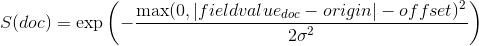where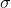is computed to assure that the score takes the value `decay` at distance `scale` from `origin`+-`offset`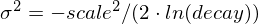See Normal decay, keyword `gauss` for graphs demonstrating the curve generated by the `gauss` function.

`exp`

Exponential decay, computed as: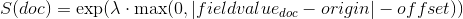where again the parameter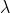is computed to assure that the score takes the value `decay` at distance `scale` from `origin`+-`offset`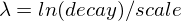See Exponential decay, keyword `exp` for graphs demonstrating the curve generated by the `exp` function.

`linear`

Linear decay, computed as: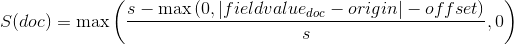.

where again the parameter `s` is computed to assure that the score takes the value `decay` at distance `scale` from `origin`+-`offset`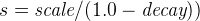In contrast to the normal and exponential decay, this function actually sets the score to 0 if the field value exceeds twice the user given scale value.

For single functions the three decay functions together with their parameters can be visualized like this (the field in this example called "age"):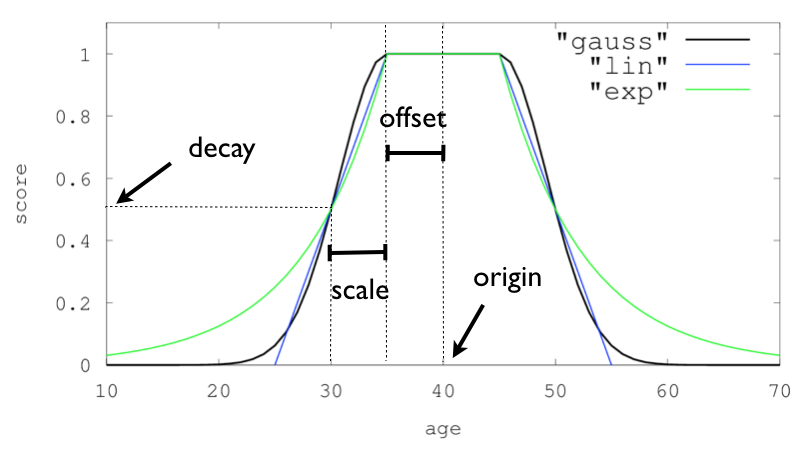#### Multi-values fieldsedit

If a field used for computing the decay contains multiple values, per default the value closest to the origin is chosen for determining the distance. This can be changed by setting `multi_value_mode`.

 `min` Distance is the minimum distance `max` Distance is the maximum distance `avg` Distance is the average distance `sum` Distance is the sum of all distances

Example:

```    "DECAY_FUNCTION": {
"FIELD_NAME": {
"origin": ...,
"scale": ...
},
"multi_value_mode": "avg"
}```

### Detailed exampleedit

Suppose you are searching for a hotel in a certain town. Your budget is limited. Also, you would like the hotel to be close to the town center, so the farther the hotel is from the desired location the less likely you are to check in.

You would like the query results that match your criterion (for example, "hotel, Nancy, non-smoker") to be scored with respect to distance to the town center and also the price.

Intuitively, you would like to define the town center as the origin and maybe you are willing to walk 2km to the town center from the hotel.
In this case your origin for the location field is the town center and the scale is ~2km.

If your budget is low, you would probably prefer something cheap above something expensive. For the price field, the origin would be 0 Euros and the scale depends on how much you are willing to pay, for example 20 Euros.

In this example, the fields might be called "price" for the price of the hotel and "location" for the coordinates of this hotel.

The function for `price` in this case would be

```"gauss": {
"price": {
"origin": "0",
"scale": "20"
}
}```
 This decay function could also be `linear` or `exp`.

and for `location`:

```"gauss": {
"location": {
"origin": "11, 12",
"scale": "2km"
}
}```
 This decay function could also be `linear` or `exp`.

Suppose you want to multiply these two functions on the original score, the request would look like this:

```response = client.search(
body: {
query: {
function_score: {
functions: [
{
gauss: {
price: {
origin: '0',
scale: '20'
}
}
},
{
gauss: {
location: {
origin: '11, 12',
scale: '2km'
}
}
}
],
query: {
match: {
properties: 'balcony'
}
},
score_mode: 'multiply'
}
}
}
)
puts response```
```res, err := es.Search(
"query": {
"function_score": {
"functions": [
{
"gauss": {
"price": {
"origin": "0",
"scale": "20"
}
}
},
{
"gauss": {
"location": {
"origin": "11, 12",
"scale": "2km"
}
}
}
],
"query": {
"match": {
"properties": "balcony"
}
},
"score_mode": "multiply"
}
}
}`)),
es.Search.WithPretty(),
)
fmt.Println(res, err)```
```GET /_search
{
"query": {
"function_score": {
"functions": [
{
"gauss": {
"price": {
"origin": "0",
"scale": "20"
}
}
},
{
"gauss": {
"location": {
"origin": "11, 12",
"scale": "2km"
}
}
}
],
"query": {
"match": {
"properties": "balcony"
}
},
"score_mode": "multiply"
}
}
}```

Next, we show how the computed score looks like for each of the three possible decay functions.

#### Normal decay, keyword `gauss`edit

When choosing `gauss` as the decay function in the above example, the contour and surface plot of the multiplier looks like this:Suppose your original search results matches three hotels :

• "Backback Nap"
• "Drink n Drive"
• "BnB Bellevue".

"Drink n Drive" is pretty far from your defined location (nearly 2 km) and is not too cheap (about 13 Euros) so it gets a low factor a factor of 0.56. "BnB Bellevue" and "Backback Nap" are both pretty close to the defined location but "BnB Bellevue" is cheaper, so it gets a multiplier of 0.86 whereas "Backpack Nap" gets a value of 0.66.

#### Exponential decay, keyword `exp`edit

When choosing `exp` as the decay function in the above example, the contour and surface plot of the multiplier looks like this:#### Linear decay, keyword `linear`edit

When choosing `linear` as the decay function in the above example, the contour and surface plot of the multiplier looks like this:### Supported fields for decay functionsedit

Only numeric, date, and geopoint fields are supported.

### What if a field is missing?edit

If the numeric field is missing in the document, the function will return 1.